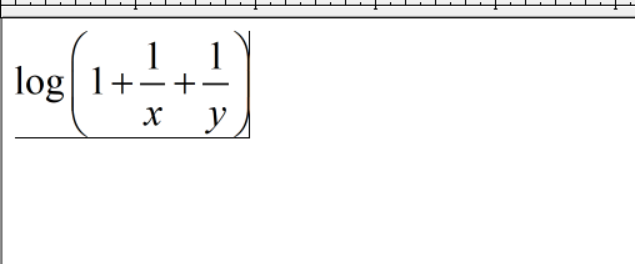# How to deal with this problem?How to use cvx to represent the following equation, where x and y are optimization variables and all optimization variables are non-negative. I have proved by strict mathematics that the representation is a convex function, but when I use cvx, I always get the error log(convex).

Use the log-sum-inv as described in How to express log(1+ sum ( 1./x ) ) in CVX? and Log( {convex} ) , which reference the Mosek Modeling cookbook section 5.2.7